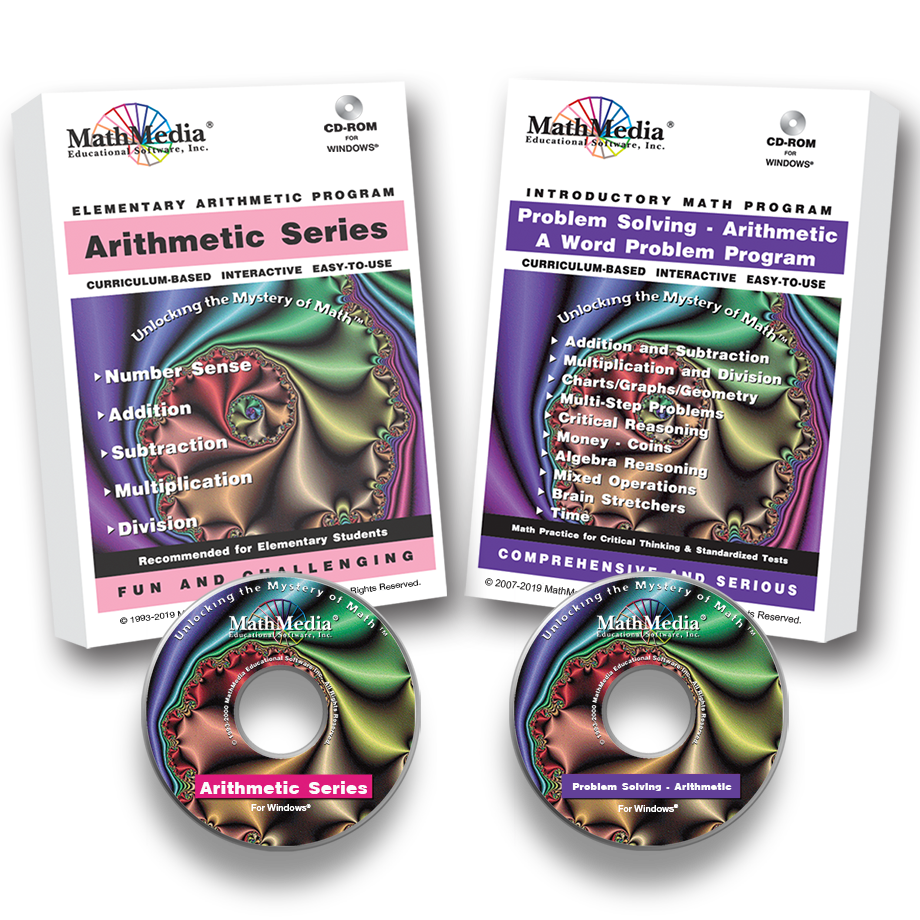# Arithmetic Series PLUS Word Problems

Regular price \$189.00 \$47.25 Sale

Purchase the 5-program "Arithmetic Series" PLUS the "Word Problems for Arithmetic".

These six elementary math programs teach the Common Core Standards in arithmetic concepts and math facts. Arithmetic skills are learned, practiced, and mastered in an easy-to-use, menu-driven learning system of instruction, practice, and assessments.

This 5-program Arithmetic Series includes:
NUMBER SENSE
SUBTRACTION
MULTIPLICATION
DIVISION

Arithmetic Series Curriculum SyllabusEach program has 10-15 learning sections with audio. Each math learning section has 20-30 interactive practice screens and concludes with a quiz. When assessments are completed, the scores are recorded on the included database. Each section has a mirror-image printable worksheet for pencil and paper work with many extras, such as brain teasers.

Word Problems for Arithmetic

Educational Testing Computer Program - This interactive arithmetic problem solving program is for elementary math students who have mastered the mechanics of the operations on whole numbers. With over 200 menu-driven practice problems (divided into 10-problem, 30 minute sections), students will be able to focus on reading the words, deciphering the meaning, and applying what they know about basic arithmetic.

These programs automatically bookmark the student's last viewed screen and will prompt the student to return to that screen upon their return.

With this 6-program package, students may navigate back and forth from the "Arithmetic Series" skills programs to this comprehensive Arithmetic Word Problems program. Learn both arithmetic skills and arithmetic problem solving necessary for success on standardized assessments.

Please note: Word problems are also scattered throughout our 5-program "Arithmetic Series" - this new arithmetic word problem program is comprehensive and strictly for practicing problem solving skills. Give students the opportunity to prepare thoroughly and comprehensively for not only standardized math tests but also to be a strong math student. "The more you know, the farther you go!"